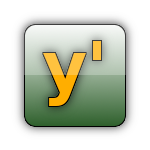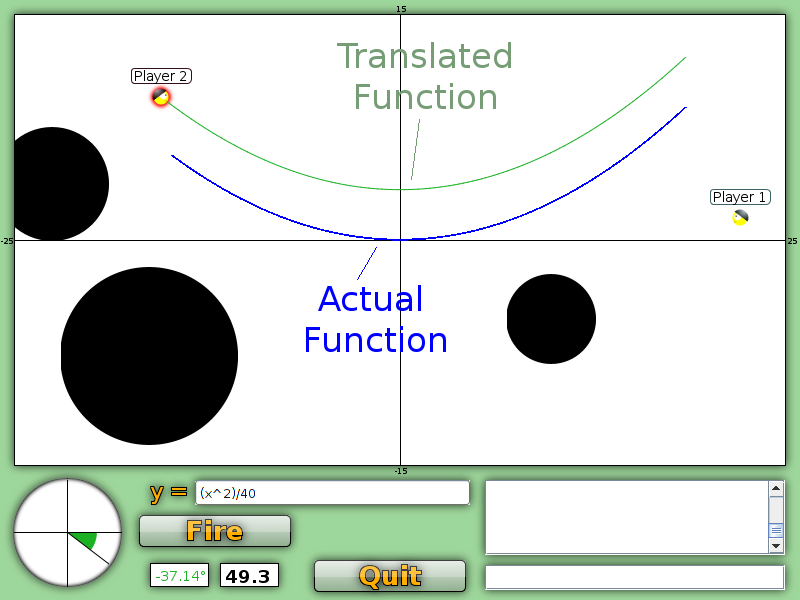# Graphwar Tutorial

Graphwar is an artillery game in which you must hit your enemies using mathematical functions. The trajectory of your shot is determined by the function you wrote, and your goal is to avoid the obstacles and your teammates and hit your enemies. The game takes place in a Cartesian Plane.## Game Modes### Normal Function

The Normal Function mode is the most basic mode. In this mode the function shot is simply the function you typed in, so the trajectory of your shot will be same trajectory as the function's graph.
However, there is a problem. The function must be shot by your soldier, but there's is no guarantee that the point where your soldier is standing belongs to the function. To solve this the function must be translated until the position of the soldier is part of the function, this is done adding a constant to the function. That means that if a function y = f(x) is typed the actual graph is actually going to be y = f(x)+c.

### First Order Differential Equation

In this mode you enter a first order differential equation instead of a function. For example:

• y' = 3*sin(x)+2
• y' = -y/3
• y' = 1/(x+y)

On this mode no constant is added to your function. Instead your soldier position is used as the initial condition to solve the differential equation and the graph fired is the actual solution.

### Second Order Differential Equation

The second order differential equation mode is very similar to the first order mode, but now you enter a second order differential equation:

• y'' = -y + y' + 2*x - 1
• y'' = 4*sin(x) + 2^x
• y'' = 1.04^(-(x+ y)^2)

To have a unique solution, a second order differential equation must have two initial conditions, the first is the soldier's position and the second is the firing angle. You can change the firing angle by pressing up and down on the keyboard. Also note that this is the only mode that the angle affects the function.

### Common PitfallsThe translation of the function have some confusing consequences. First, any constant added to your function is irrelevant to the result. For example, the functions y = 2*x + 3, y = 2*x - 8 and y = 2*x yield the exact same graph in the game.

Other confusing fact is related to the fact that the x axis limits on the game are -25 and +25 and the y axis limits are -15 and 15. That means functions can get very big. For example the function y = x^2 has the value 100 when x equals 10. That means this function will hit the ceiling of the game very fast. If your soldier is positioned on a position where x is -15 this function will be very very steep, it will most likely appear as a straight line up or down. Remember that a huge constant will have to be added to this function, so the result is something very different from what you might be expecting. This problem can be solved by scaling the function appropriately, the function y = (x^2)/50 will produce a nice looking parabola.

Another thing that may confuse you is that your soldiers will always be standing on negative values for x. Your team is located to the left of the y axis, so that is expected and it means functions like y = sqrt(x) will not like you and will explode immediately. You should try something like y = sqrt(abs(x)).
As was just pointed out functions may explode spontaneously. That means it had an invalid value at that point, a square root of a negative number or a function that gets vertical at a point will explode. Another possible reason for a function to explode is that it is too long, a sine with a high frequency may reach the maximum function length allowed and spontaneously explode.

### Function Syntax

• Variables

• x
• y
• y'

• Operators

• +
• -
• /
• *
• ^

• Functions

• sqrt()
• log()
• ln()
• abs()
• sin()
• cos()
• tan()
• exp()

• Other Examples

• y = ((x-3)^2)/20
• y = ln(abs(x))
• y = sin(x/20)*5
• y' = 1.2^x
• y'' = (1.2^(-(x+3)^2))*(20*(-y))

Using lots of parentheses is recommended to avoid misinterpretation, for example y = 1/x+2 is going to be understood as (1/x) + 2, you should use 1/(x+2).

### Chat Commands

The available commands are:

• -skip : If everyone playing uses this command, the current map is skipped and a new one is generated.
• -sayfunc : If you use this you are going to see on your chat the function that everyone else is using.
• -stopsayfunc : This will stop the functions from appearing on your chat after you used -sayfunc.
• -shownext : This will highlight the next soldier to play for each player with a dark circle. This is useful to plan functions ahead of time.
• -stopshownext : Stops showing the next soldier to play.

Just type them on the game chat to use them.